Courses

# Boundary Value Problems(Part- 1) - Electrostatics, Electromagnetic Theory, CSIR-NET Physical Science Physics Notes | EduRev

## Physics for IIT JAM, UGC - NET, CSIR NET

Created by: Akhilesh Thakur

## Physics : Boundary Value Problems(Part- 1) - Electrostatics, Electromagnetic Theory, CSIR-NET Physical Science Physics Notes | EduRev

The document Boundary Value Problems(Part- 1) - Electrostatics, Electromagnetic Theory, CSIR-NET Physical Science Physics Notes | EduRev is a part of the Physics Course Physics for IIT JAM, UGC - NET, CSIR NET.
All you need of Physics at this link: Physics

Example: Dielectric Filled Parallel Plates Consider two infinite, parallel conducting plates, spaced a distance d apart. The region between the plates is filled with a dielectric ε .  Say a voltage V0 is placed across these plates.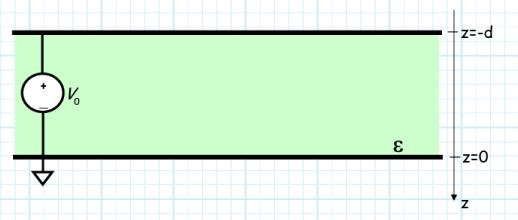Q: What electric potential field V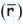, electric field Eand charge density ρsis produced by this situation?

A: We must solve a boundary value problem !  We must find solutions that:

a) Satisfy the differential equations of electrostatics (e.g., Poisson’s, Gauss’s).

b)  Satisfy the electrostatic boundary conditions.

Q: Yikes! Where do we even start ?
A:
We might start with the electric potential field V, since it is a scalar field. a)  The electric potential function must satisfy Poisson’s equation: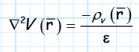b) It must also satisfy the boundary conditions:

V z =−d =V              V z = 0 = 0

Consider first the dielectric region ( −d <z< 0 ).

Since the region is a dielectric, there is no free charge, and:

ρv=0

Therefore, Poisson’s equation reduces to Laplace’s equation: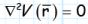This problem is greatly simplified, as it is evident that the solution Vis independent of coordinates x and y .  In other words, the electric potential field will be a function of coordinate z only: V=V (z )

This make the problem much easier!  Laplace’s equation becomes: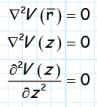Integrating both sides of Laplace’s equation, we get: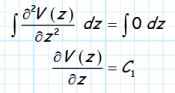And integrating again we find: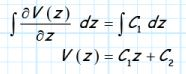We find that the equation V (z ) =C1z + C2 will satisfy Laplace’s equation  (try it!).  We must now apply the boundary conditions to determine the value of constants Cand C2.

We know that the value of the electrostatic potential at every point on the top (z =-d) plate is  V (-d)=V0, while the electric potential on the bottom plate (z =0) is zero (V (0) =0 ).  Therefore: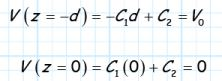Two equations and two unknowns (C1 and C2)! Solving for C1 and C2 we get: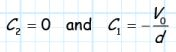and therefore, the electric potential field within the dielectric is found to be: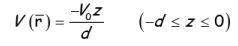Before we proceed, let’s do a sanity check!

In other words, let’s evaluate our answer at z = 0 and z = -d, to make sure our result is correct: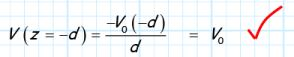and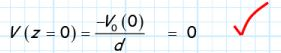Now, we can find the electric field within the dielectric by taking the gradient of our result: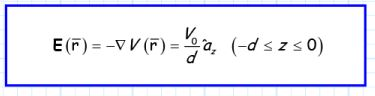And thus we can easily determine the electric flux density by multiplying by the dielectric of the material: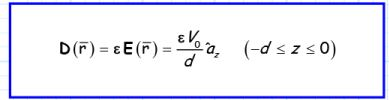Finally, we need to determine the charge density that actually created these fields!

Q: Charge density !?!  I thought that we already determined that the charge density ρvis equal to zero?

A: We know that the free charge density within the dielectric is zero—but there must be charge somewhere, otherwise there would be no fields!

Recall that we found that at a conductor/dielectric interface, the surface charge density on the conductor is related to the electric flux density in the dielectric as: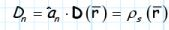First, we find that the electric flux density on the bottom surface of the top conductor (i.e., at z = −d ) is: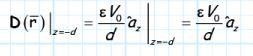For every point on bottom surface of the top conductor, we find that the unit vector normal to the conductor is: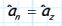Therefore, we find that the surface charge density on the bottom surface of the top conductor is: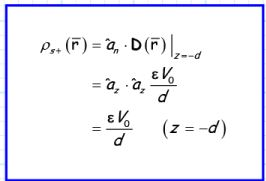Likewise, we find the unit vector normal to the top surface of the bottom conductor is (do you see why):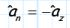Therefore, evaluating the electric flux density on the top surface of the bottom conductor (i.e., z = 0 ), we find: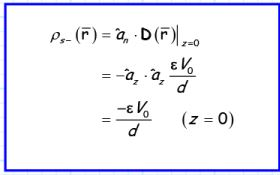We should note several things about these solutions:

1.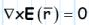2.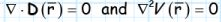3. DandEare normal to the surface of the conductor (i.e., their tangential components are equal to zero).

4. The electric field is precisely the same as that given by using superposition and eq. 4.20 in section 4-5!

I.E.: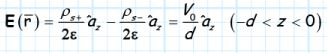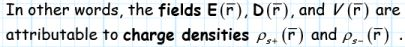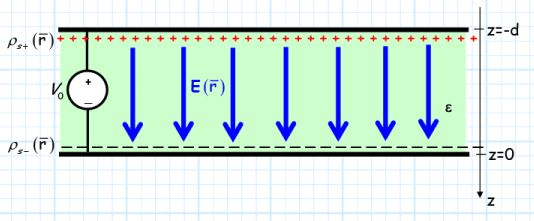Example: Charge Filled Parallel Plates

Consider now a problem similar to the previous example (i.e., dielectric filled parallel plates), with the exception that the space between the infinite, conducting parallel plates is filled with free charge, with a density: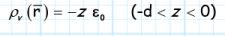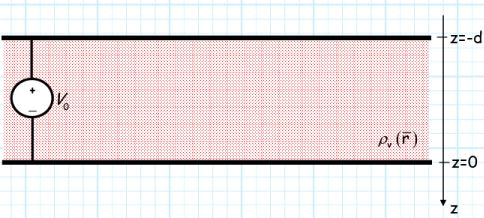Q: How do we determine the fields within the parallel plates for this problem?

A: Same as before!  However, since the charge density between the plates is not equal to zero, we recognize that the electric potential field must satisfy Poisson’s equation: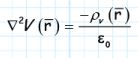For the specific charge density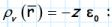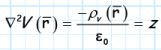Since both the charge density and the plate geometry are independent of coordinates x and y, we know the electric potential field will be a function of coordinate z only (i.e., V=V (z) ).

Therefore, Poisson’s equation becomes: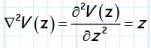We can solve this differential equation by first integrating both sides: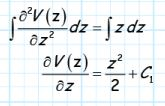And then integrating a second time: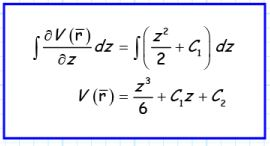Note that this expression for Vsatisfies Poisson’s equation for this case.  The question remains, however: what are the values of constants C1 and C?

We find them in the same manner as before—boundary conditions!

Note the boundary conditions for this problem are: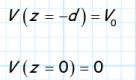Therefore, we can construct two equations with two unknowns: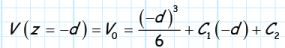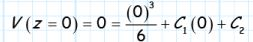It is evident that C2 = 0, therefore constant C1 is: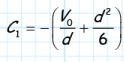The electric potential field between the two plates is therefore: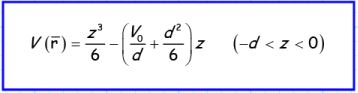Performing our sanity check, we find: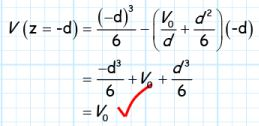and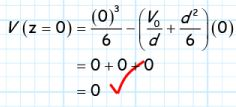From this result, we can determine the electric field E, the electric flux density D, and the surface charge density ρ s, as before.
Note, however, that the permittivity of the material between the plates is ε0, as the “dielectric” between the plates is freespace.

Offer running on EduRev: Apply code STAYHOME200 to get INR 200 off on our premium plan EduRev Infinity!

159 docs

,

,

,

,

,

,

,

,

,

,

,

,

,

,

,

,

,

,

,

,

,

,

,

,

,

,

,

;